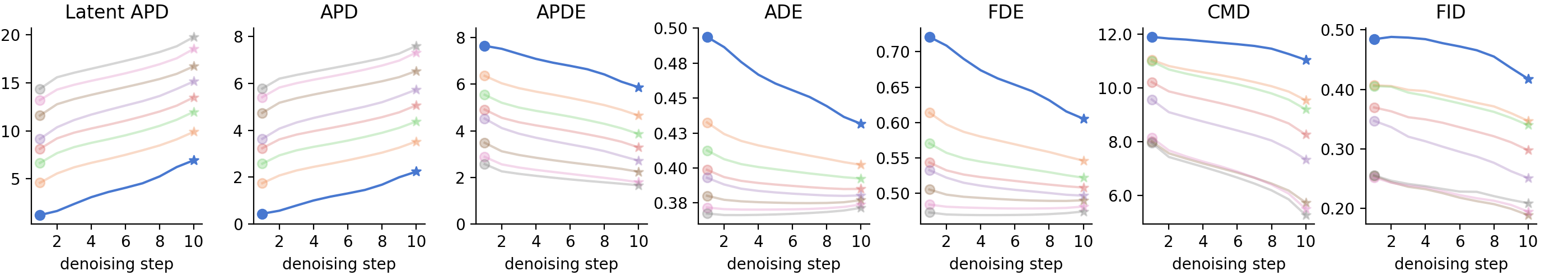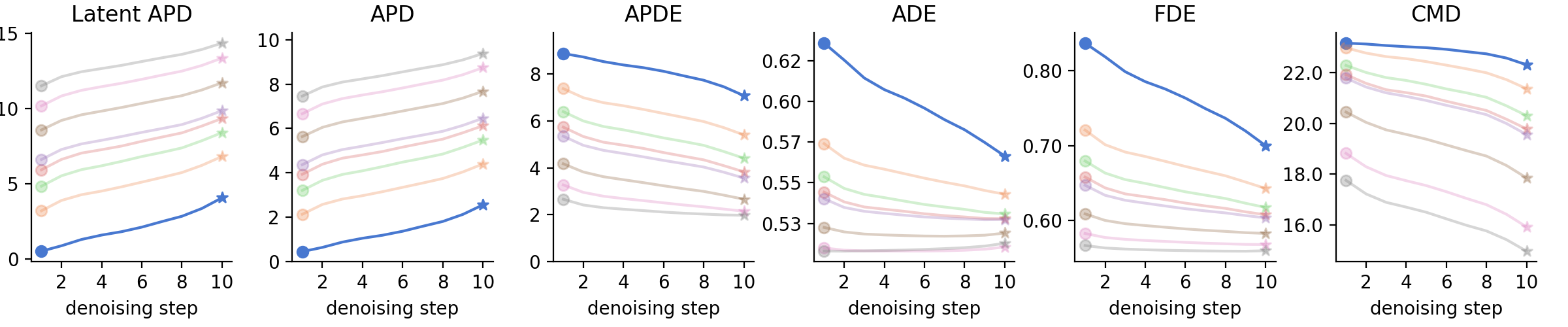# BeLFusion: Latent Diffusion for Behavior-Driven Human Motion Prediction

ICCV 2023

### Abstract

Stochastic human motion prediction (HMP) has generally been tackled with generative adversarial networks and variational autoencoders. Most prior works aim at predicting highly diverse movements in terms of the skeleton joints’ dispersion. This has led to methods predicting fast and motion-divergent movements, which are often unrealistic and incoherent with past motion. Such methods also neglect contexts that need to anticipate diverse low-range behaviors, or actions, with subtle joint displacements. To address these issues, we present BeLFusion, a model that, for the first time, leverages latent diffusion models in HMP to sample from a latent space where behavior is disentangled from pose and motion. As a result, diversity is encouraged from a behavioral perspective. Thanks to our behavior coupler’s ability to transfer sampled behavior to ongoing motion, BeLFusion’s predictions display a variety of behaviors that are significantly more realistic than the state of the art. To support it, we introduce two metrics, the Area of the Cumulative Motion Distribution, and the Average Pairwise Distance Error, which are correlated to our definition of realism according to a qualitative study with 126 participants. Finally, we prove BeLFusion’s generalization power in a new cross-dataset scenario for stochastic HMP.

### Motivations

Most prior stochastic works focus on predicting a highly diverse distribution of motions. Such diversity has been traditionally defined and evaluated in the coordinate space. This definition biases research toward models that generate fast and motion-divergent motions (see Fig. in the left). Although there are scenarios where predicting low-speed diverse motion is important, this is discouraged by prior techniques. For example, in assistive robotics, anticipating behaviors (i.e., actions) like whether the interlocutor is about to shake your hand or scratch their head might be crucial for preparing the robot’s actuators on time. In a surveillance scenario, a foreseen noxious behavior might not differ much from a well-meaning one when considering only the poses along the motion sequence. We argue that this behavioral perspective is paramount to build next-generation stochastic HMP models.

BeLFusion, by building a latent space where behavior is disentangled from poses and motion, detaches diversity from the traditional coordinate-based perspective and promotes it from a behavioral viewpoint.
Results from prior diversity-centric works often suffer from a tradeoff that has been persistently overlooked: predicted motion looks unnatural when observed following the motion of the immediate past. The strong diversity regularization techniques employed often produce abrupt speed changes or direction discontinuities. We argue that consistency with the immediate past is a requirement for prediction plausibility.

Figure below shows the evolution of 10 superimposed predictions along time in two actions from H36M (sitting down, and giving directions), and two datasets from AMASS (DanceDB, and GRAB). First, the acceleration of GSPS and DivSamp at the beginning of the prediction leads to extreme poses very fast, abruptly transitioning from the observed motion. Second, it shows the capacity of BeLFusion to adapt the diversity predicted to the context. For example, the diversity of motion predicted while giving directions focuses on the upper body, and does not include holistic extreme poses. Interestingly, when just sitting, the predictions include a wider range of full-body movements like laying down, or bending over. A similar context fitting is observed in the AMASS cross-dataset scenario. For instance, BeLFusion correctly identifies that the diversity must target the upper body in the GRAB dataset, or the arms while doing a dance step.

### Architecture

A latent diffusion model conditioned on an encoding $$x=c$$ of the observation, $$\mathbf{X}$$, progressively denoises a sample from a zero-mean unit variance multivariate normal distribution into a behavior code. Then, the behavior coupler $$\mathcal{B}_{\phi}$$ decodes the prediction by transferring the sampled behavior to the target motion, $$\mathbf{x}_{m}$$. In our implementation, $$f_{\Phi}$$ is a conditional U-Net with cross-attention, and $$h_{\lambda}$$, $$g_{\alpha}$$, and $$\mathcal{B}_{\phi}$$ are one-layer recurrent neural networks.

### Implicit diversity loss

$$\underset{k}{\min}\; \mathcal{L}_{lat}(\mathbf{X}, \mathbf{Y}_{e}^k) + \lambda \; \underset{k}{\min}\; \mathcal{L}_{rec}(\mathbf{X}, \mathbf{Y}_{e}^k)$$
Regularization relaxation value: k=1
##### Human3.6M##### AMASSRegularization relaxation usually leads to out-of-distribution predictions. This is often solved by employing additional complex techniques like pose priors, or bone-length losses that regularize the other predictions. BeLFusion can dispense with it due to mainly two reasons: 1) Denoising diffusion models are capable of faithfully capturing a greater breadth of the training distribution than GANs or VAEs; 2) The variational training of the behavior coupler makes it more robust to errors in the predicted behavior code.

In general, increasing k enhances the samples' diversity, accuracy, and realism. For k < 5, going through the whole chain of denoising steps boosts accuracy. However, for k > 5, further denoising only boosts diversity- and realism-wise metrics (APD, CMD, FID), and makes the fast single-step inference extremely accurate.